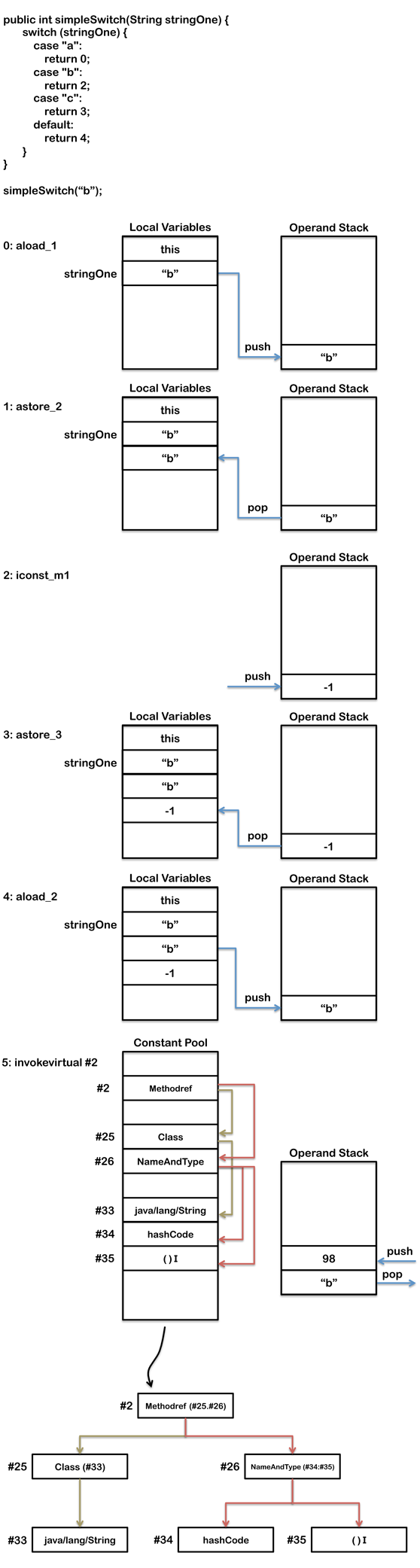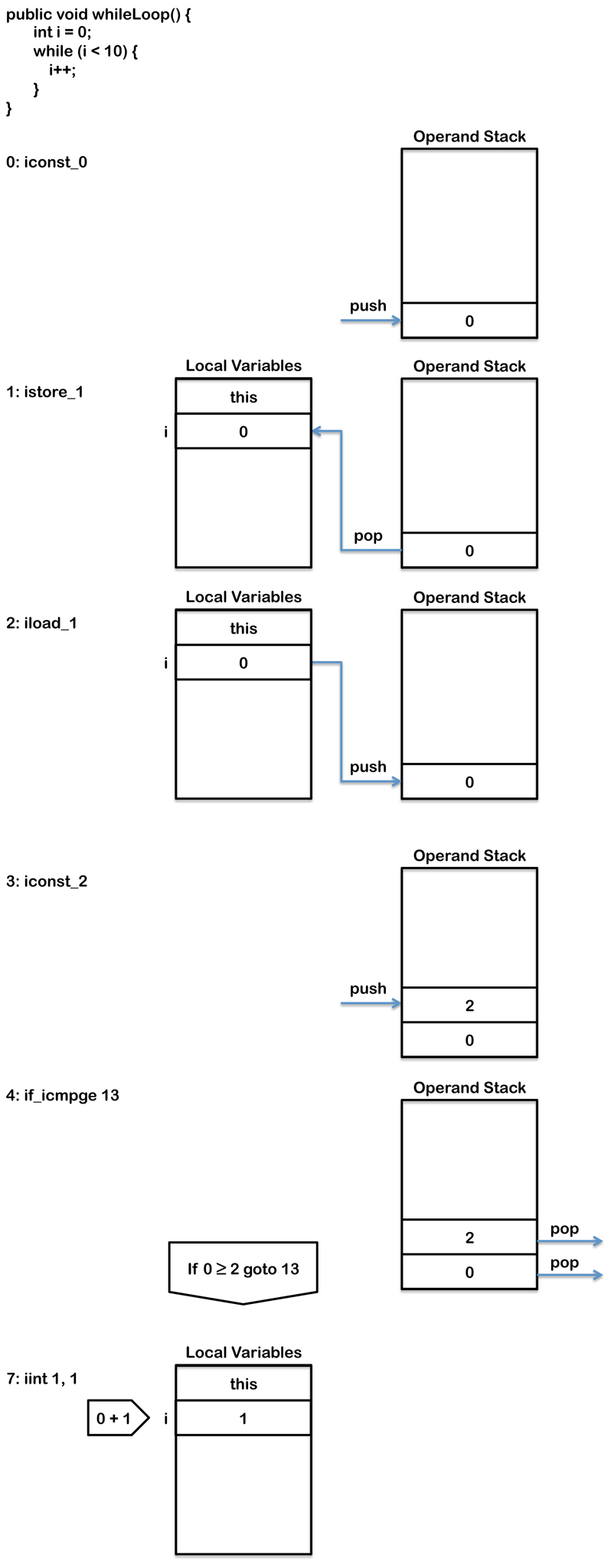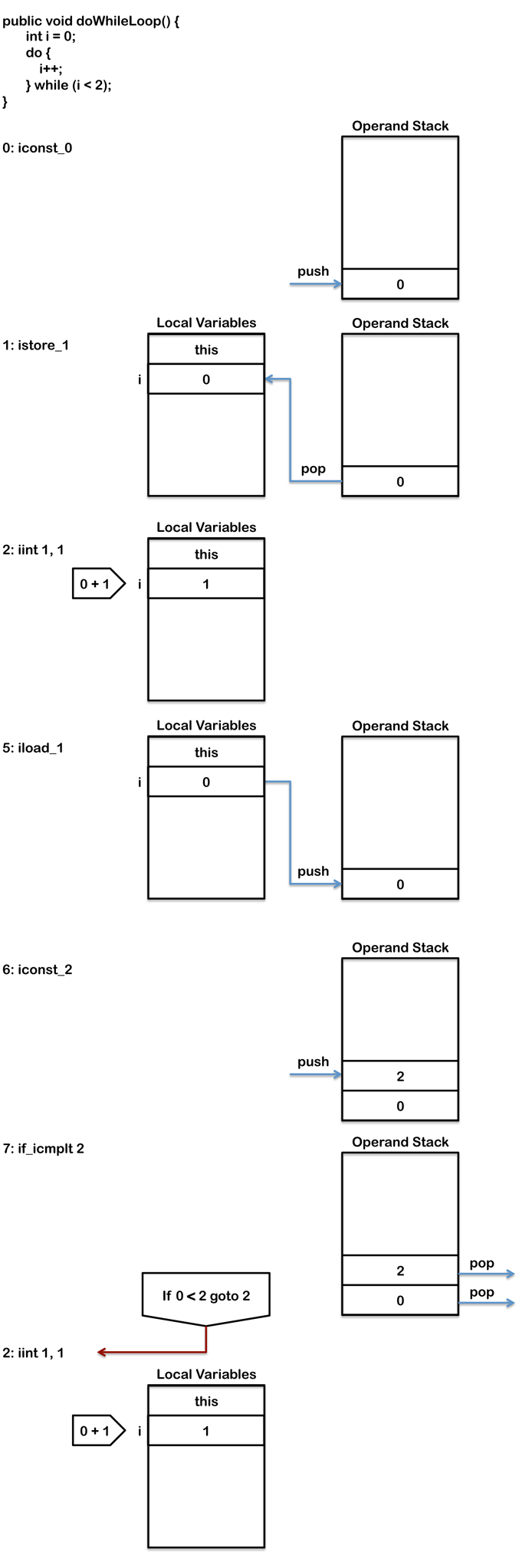# Java字节码浅析（三）

 `01` `public` `int` `simpleSwitch(String stringOne) {`
 `02` `    ``switch` `(stringOne) {`
 `03` `        ``case` `"a"``:`
 `04` `            ``return` `0``;`
 `05` `        ``case` `"b"``:`
 `06` `            ``return` `2``;`
 `07` `        ``case` `"c"``:`
 `08` `            ``return` `3``;`
 `09` `        ``default``:`
 `10` `            ``return` `4``;`
 `11` `    ``}`
 `12` `}`

 `01` `0``: aload_1`
 `02` ` ``1``: astore_2`
 `03` ` ``2``: iconst_m1`
 `04` ` ``3``: istore_3`
 `05` ` ``4``: aload_2`
 `06` ` ``5``: invokevirtual #``2`                  `// Method java/lang/String.hashCode:()I`
 `07` ` ``8``: tableswitch   {`
 `08` `         ``default``: ``75`
 `09` `             ``min: ``97`
 `10` `             ``max: ``99`
 `11` `              ``97``: ``36`
 `12` `              ``98``: ``50`
 `13` `              ``99``: ``64`
 `14` `       ``}`
 `15` `36``: aload_2`
 `16` `37``: ldc           #``3`                  `// String a`
 `17` `39``: invokevirtual #``4`                  `// Method java/lang/String.equals:(Ljava/lang/Object;)Z`
 `18` `42``: ifeq          ``75`
 `19` `45``: iconst_0`
 `20` `46``: istore_3`
 `21` `47``: ``goto`          `75`
 `22` `50``: aload_2`
 `23` `51``: ldc           #``5`                  `// String b`
 `24` `53``: invokevirtual #``4`                  `// Method java/lang/String.equals:(Ljava/lang/Object;)Z`
 `25` `56``: ifeq          ``75`
 `26` `59``: iconst_1`
 `27` `60``: istore_3`
 `28` `61``: ``goto`          `75`
 `29` `64``: aload_2`
 `30` `65``: ldc           #``6`                  `// String c`
 `31` `67``: invokevirtual #``4`                  `// Method java/lang/String.equals:(Ljava/lang/Object;)Z`
 `32` `70``: ifeq          ``75`
 `33` `73``: iconst_2`
 `34` `74``: istore_3`
 `35` `75``: iload_3`
 `36` `76``: tableswitch   {`
 `37` `         ``default``: ``110`
 `38` `             ``min: ``0`
 `39` `             ``max: ``2`
 `40` `               ``0``: ``104`
 `41` `               ``1``: ``106`
 `42` `               ``2``: ``108`
 `43` `       ``}`
 `44` `104``: iconst_0`
 `45` `105``: ireturn`
 `46` `106``: iconst_2`
 `47` `107``: ireturn`
 `48` `108``: iconst_3`
 `49` `109``: ireturn`
 `50` `110``: iconst_4`
 `51` `111``: ireturn`

 `01` `Constant pool:`
 `02` `  ``#``2` `= Methodref          #``25``.#``26`        `//  java/lang/String.hashCode:()I`
 `03` `  ``#``3` `= String             #``27`            `//  a`
 `04` `  ``#``4` `= Methodref          #``25``.#``28`        `//  java/lang/String.equals:(Ljava/lang/Object;)Z`
 `05` `  ``#``5` `= String             #``29`            `//  b`
 `06` `  ``#``6` `= String             #``30`            `//  c`
 `07`
 `08` ` ``#``25` `= Class              #``33`            `//  java/lang/String`
 `09` ` ``#``26` `= NameAndType        #``34``:#``35`        `//  hashCode:()I`
 `10` ` ``#``27` `= Utf8               a`
 `11` ` ``#``28` `= NameAndType        #``36``:#``37`        `//  equals:(Ljava/lang/Object;)Z`
 `12` ` ``#``29` `= Utf8               b`
 `13` ` ``#``30` `= Utf8               c`
 `14`
 `15` ` ``#``33` `= Utf8               java/lang/String`
 `16` ` ``#``34` `= Utf8               hashCode`
 `17` ` ``#``35` `= Utf8               ()I`
 `18` ` ``#``36` `= Utf8               equals`
 `19` ` ``#``37` `= Utf8               (Ljava/lang/Object;)Z``01` `public` `int` `simpleSwitch(String stringOne) {`
 `02` `    ``switch` `(stringOne) {`
 `03` `        ``case` `"FB"``:`
 `04` `            ``return` `0``;`
 `05` `        ``case` `"Ea"``:`
 `06` `            ``return` `2``;`
 `07` `        ``default``:`
 `08` `            ``return` `4``;`
 `09` `    ``}`
 `10` `}`

 `01` `0``: aload_1`
 `02` ` ``1``: astore_2`
 `03` ` ``2``: iconst_m1`
 `04` ` ``3``: istore_3`
 `05` ` ``4``: aload_2`
 `06` ` ``5``: invokevirtual #``2`                  `// Method java/lang/String.hashCode:()I`
 `07` ` ``8``: lookupswitch  {`
 `08` `         ``default``: ``53`
 `09` `           ``count: ``1`
 `10` `            ``2236``: ``28`
 `11` `    ``}`
 `12` `28``: aload_2`
 `13` `29``: ldc           #``3`                  `// String Ea`
 `14` `31``: invokevirtual #``4`                  `// Method java/lang/String.equals:(Ljava/lang/Object;)Z`
 `15` `34``: ifeq          ``42`
 `16` `37``: iconst_1`
 `17` `38``: istore_3`
 `18` `39``: ``goto`          `53`
 `19` `42``: aload_2`
 `20` `43``: ldc           #``5`                  `// String FB`
 `21` `45``: invokevirtual #``4`                  `// Method java/lang/String.equals:(Ljava/lang/Object;)Z`
 `22` `48``: ifeq          ``53`
 `23` `51``: iconst_0`
 `24` `52``: istore_3`
 `25` `53``: iload_3`
 `26` `54``: lookupswitch  {`
 `27` `         ``default``: ``84`
 `28` `           ``count: ``2`
 `29` `               ``0``: ``80`
 `30` `               ``1``: ``82`
 `31` `    ``}`
 `32` `80``: iconst_0`
 `33` `81``: ireturn`
 `34` `82``: iconst_2`
 `35` `83``: ireturn`
 `36` `84``: iconst_4`
 `37` `85``: ireturn`

### 循环语句

if-else 和 switch 这些条件流程控制语句都是先通过一条指令比较两个值，然后跳转到某个分支去执行。

for 循环和 while 循环这些语句也类似，只不过它们通常都包含一个 goto 指令，使得字节码能够循环执行。do-while 循环则不需要 goto 指令，因为它们的条件判断指令是放在循环体的最后来执行。

####while 循环

while 循环包含条件跳转指令比如 if_icmpge 或者 if_icmplt（前面有介绍）以及 goto 指令。如果判断条件不满足的话，会跳转到循环体后的第一条指令继续执行，循环结束（译注：这里判断条件和代码中的正好相反，如代码中是 i<2，字节码内是 i>=2，从字节码的角度看，是满足条件后循环中止）。循环体的末尾是一条 goto 指令，它会跳转到循环开始的地方继续执行，直到分支跳转的条件满足才终止。

 `1` `public` `void` `whileLoop() {`
 `2` `    ``int` `i = ``0``;`
 `3` `    ``while` `(i < ``2``) {`
 `4` `        ``i++;`
 `5` `    ``}`
 `6` `}`

 `1` `0``: iconst_0`
 `2` `1``: istore_1`
 `3` `2``: iload_1`
 `4` `3``: iconst_2`
 `5` `4``: if_icmpge ``13`
 `6` `7``: iinc ``1``, ``1`
 `7` `10``: ``goto` `2`
 `8` `13``: ``return`

if_icmpge 指令会判断局部变量区中的 1 号位的变量（也就是 i，译注：局部变量区从 0 开始计数，第 0 位是 this) 是否大于等于 2，如果不是继续执行，如果是的话跳转到 13 行处，结束循环。goto 指令使得循环可以继续执行，直到条件判断为真，这个时候会跳转到紧挨着循环体后边的 return 指令处。iinc 是少数的几条能直接更新局部变量区里的变量的指令之一，它不用把值压到操作数栈里面就能直接进行操作。这里 iinc 指令把第 1 个局部变量（译注：第 0 个是 this）自增 1。for 循环和 while 循环在字节码里的格式是一样的。这并不奇怪，因为每个 while 循环都可以很容易改写成一个 for 循环。比如上面的 while 循环就可以改写成下面的 for 循环，当然了它们输出的字节码也是一样的：

 `1` `public` `void` `forLoop() {`
 `2` `    ``for``(``int` `i = ``0``; i < ``2``; i++) {`
 `3`
 `4` `    ``}`
 `5` `}`

####do-while 循环

do-while 循环和 for 循环，while 循环非常类似，除了一点，它是不需要 goto 指令的，因为条件跳转指令在循环体的末尾，可以用它来跳转回循环体的起始处。

 `1` `public` `void` `doWhileLoop() {`
 `2` `    ``int` `i = ``0``;`
 `3` `    ``do` `{`
 `4` `        ``i++;`
 `5` `    ``} ``while` `(i < ``2``);`
 `6` `}`

 `1` `0``: iconst_0`
 `2` `1``: istore_1`
 `3` `2``: iinc          ``1``, ``1`
 `4` `5``: iload_1`
 `5` `6``: iconst_2`
 `6` `7``: if_icmplt    ``2`
 `7` `10``: ``return`